Add Labels and Text to Matplotlib Plots: Annotation Examples

# Add Labels and Text to Matplotlib Plots: Annotation Examples

Last updated:

Used matplotlib version 3.x. View all code on this notebook

See all options you can pass to plt.text here: valid keyword args for plt.txt

Use plt.text(<x>, <y>, <text>):

import matplotlib.pyplot as plt
import numpy as np

plt.clf()

# using some dummy data for this example
xs = np.arange(0,10,1)
ys = np.random.normal(loc=2.0, scale=0.8, size=10)

plt.plot(xs,ys)

# text is left-aligned
plt.text(2,4,'This text starts at point (2,4)')

# text is right-aligned
plt.text(8,3,'This text ends at point (8,3)',horizontalalignment='right')

plt.show()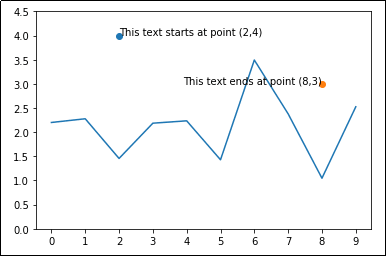Text can be left- or right-aligned

## Add labels to line plots

Again, zip together the data (x and y) and loop over it, call plt.annotate(<label>, (<x>,<y>))

import matplotlib.pyplot as plt
import numpy as np

plt.clf()

# using some dummy data for this example
xs = np.arange(0,10,1)
ys = np.random.normal(loc=3, scale=0.4, size=10)

# 'bo-' means blue color, round points, solid lines
plt.plot(xs,ys,'bo-')

# zip joins x and y coordinates in pairs
for x,y in zip(xs,ys):

label = "{:.2f}".format(y)

plt.annotate(label, # this is the text
(x,y), # these are the coordinates to position the label
textcoords="offset points", # how to position the text
xytext=(0,10), # distance from text to points (x,y)
ha='center') # horizontal alignment can be left, right or center

plt.show()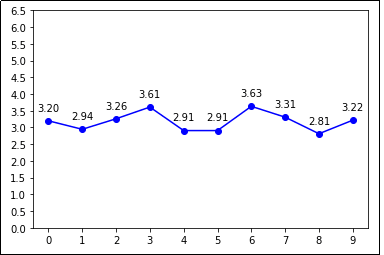Labels contain the value of Y, with 2 decimal places

## Add labels to bar plots

Loop over the arrays (xs and ys) and call plt.annotate(<label>, <coords>):

import matplotlib.pyplot as plt
import numpy as np

plt.clf()

# using some dummy data for this example
xs = np.arange(0,10,1)
ys = np.random.normal(loc=3, scale=0.4, size=10)

plt.bar(xs,ys)

# zip joins x and y coordinates in pairs
for x,y in zip(xs,ys):

label = "{:.2f}".format(y)

plt.annotate(label, # this is the text
(x,y), # these are the coordinates to position the label
textcoords="offset points", # how to position the text
xytext=(0,10), # distance from text to points (x,y)
ha='center') # horizontal alignment can be left, right or center

plt.show()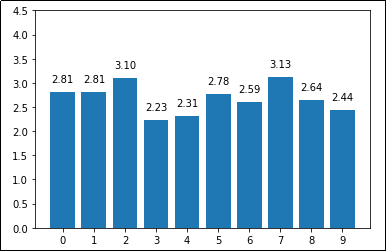As created with the above code

## Add labels to points in scatter plots

Loop over the data arrays (x and y) and call plt.annotate(<label>, <coords>) using the value itself as label:

import matplotlib.pyplot as plt
import numpy as np

# using some dummy data for this example
xs = np.random.randint( 0, 10, size=10)
ys = np.random.randint(-5, 5,  size=10)

# plot the points
plt.scatter(xs,ys)

# zip joins x and y coordinates in pairs
for x,y in zip(xs,ys):

label = f"({x},{y})"

plt.annotate(label, # this is the text
(x,y), # these are the coordinates to position the label
textcoords="offset points", # how to position the text
xytext=(0,10), # distance from text to points (x,y)
ha='center') # horizontal alignment can be left, right or center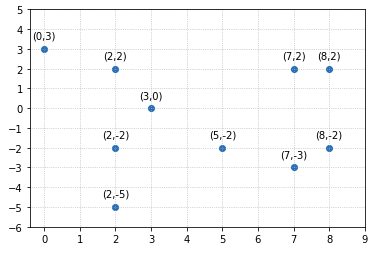As created by the above code

This is useful if you have subplots (more examples here)

To add text to an individual Axes object just call ax.annotate(<text>, <xy_coords>):

import matplotlib.pyplot as plt
import numpy as np

# generate sample data to plot
x = np.linspace(0.0,100,50)
y = np.random.uniform(low=0,high=10,size=50)

# get references to the 2 axes with plt.subplots()
fig, (ax1, ax2) = plt.subplots(1,2)

ax1.bar(x,y)

# add text to the axes on the left only
ax1.annotate('Some text in axes ax1',
(40, 8), # these are the coordinates to position the label
color='red') # you can pass any extra params too

ax2.bar(x,y)
# add text to the other axes (on the right)
ax2.annotate('More text in axes ax2',
(40, 8), # these are the coordinates to position the label
color='black',
size=16)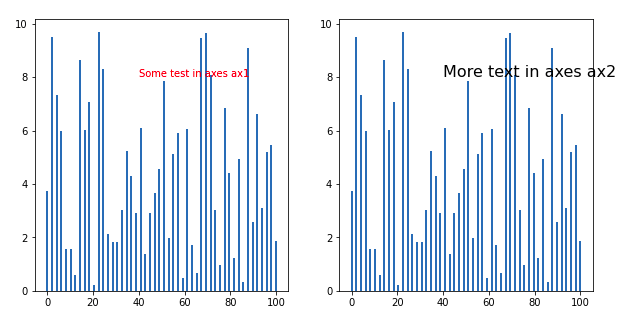Text added individually to each Axes instance,
(generated with plt.subplots())

### String tick labels

You want to position text in the plot but your plot also uses string tick labels, so you can't just use annotate('some-text', ('a', 4)) because you need actual integer x,y coordinates.

import matplotlib.pyplot as plt
import numpy as np

# generate sample data for this example
xs = [1,2,3,4,5,6,7,8,9,10,11,12]
ys = np.random.normal(loc=3.0,size=12)
labels = ['jan','feb','mar','apr','may','jun','jul','aug','sept','oct','nov','dec']

# plot
plt.bar(xs,ys)

# apply custom tick labels
plt.xticks(xs,labels)

# this is the x-axis label you want to write the text for
string_label = "feb"

# figure out which x-axis tick corresponds to that
label_index = labels.index(string_label)
xs_index    = xs[label_index]

# use xs_index to position the text
plt.annotate("foo-bar",
(xs_index,4)) # these are the coordinates to position the label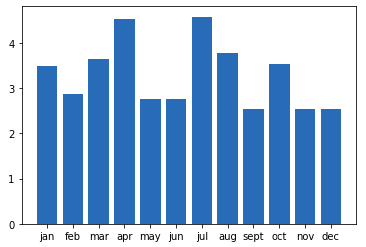BEFORE: source image without text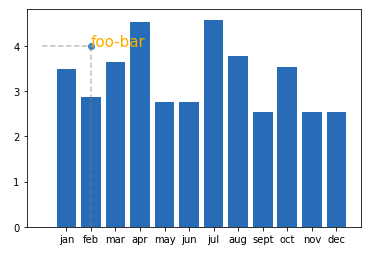AFTER: you added some text positioned
exactly at what would be ('feb',4) coordinates